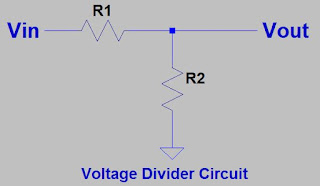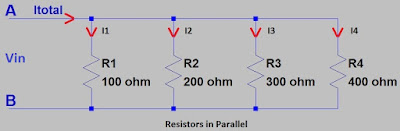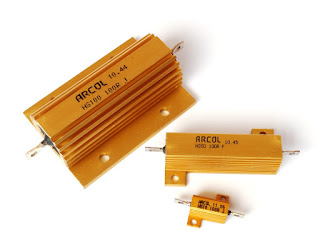# Resistor and Working of Resistor

### Resistor

A resistor is a passive component which opposes the movement of current across it in other words; it limits or controls the flow of electrical current in an electronic circuit. Apart from current control in the circuit, resistors are used for; Adjustment of signal stages, voltage divider, to bias the device like transistors, for transmission line terminations, resistive loads, etc.Resistor

### Resistor Symbol

Below image shows symbol of both fixed and variable type resistors.Resistor Symbol
Resistors are classified as; fixed and the variable type.

The fixed resistors have a fixed resistance value and this value is not adjustable. Fixed resistors have resistances which changes slightly with change in temperature, time or by operative voltage.

The variable resistors have a variable resistance value and this value is adjustable. These types of resistors can be used to amend circuit elements like amending volume or a varying the lamp intensity. Sometimes these resistors used as sensing component for heat, light, etc.

### Resistor Working

Below points presents details for resistor working;

A resistor resists current because flowing electrons strike with atoms in the resistor and make them vibrate. By this, electrical energy is changed to heat energy. The quantity by which a resistor resists the flow of charge is measured as resistance “R”.

#### Resistor works according to Ohm's law

Basic principle of working of resistor or in other words behavior of resistor working depends on the Ohm’s law. As we know Ohm’s law states that; V = I / R.

Let’s explain this; as per Ohm's law the voltage (V) through a resistor is proportional to the current (I), where the constant of proportionality is the resistance (R) i.e. the electrical resistance of the conductor is constant.

So, in simple word’s we can say that, with the additional voltage applied to a resistor the extra current flows through it.

Let’s see an example; If a 100 ohm resistor is connected across the +ve and –ve terminals of a 24V power supply, then a current of 12 / 300 = 0.24A will flow through that resistor.

The resistor causes delay i.e. potential drop to flow of current, we can understand this by an example of a pipe through which the water flows. See the image at below;Water flow in the pipe
Here the thickness of the pipe signifies the resistance. The narrow the pipe it’s tough for the water to get through the pipe and therefore larger the resistance. If you make the pipe size double, then the flow rate doubles i.e. if less resistance then more current will pass.

#### Series and parallel resistors

Resistor can be used to change a voltage to a current or a vice-versa. By connecting resistors in series or in parallel you can efficiently control the current. Let see in detail;

Resistors in series

Resistors in “Series” means, they are connected together in daisy form i.e. in a particular single line. Entire current passing across the first resistor must also pass through the second resistor and then third and so on, as there’s no other way for flow of current. Current flow has only one path i.e. resistors in series have a common current flow across them.

Below schematic shows that the resistors R1, R2, R3 and R4 are connected in series, in between points A and B and a common current (I) passing through them;Resistors in Series
Here the total current that passing across these resistors in series will be the similar at any point.
Itotal = IR1 = IR2 = IR3 = IR4

Here the total resistance is the addition of all the individual resistance of the resistors in series.
Rtotal = R1 + R2 + R3 + R4 = 100 ohm + 200 ohm + 300 ohm + 400 ohm = 1k ohm

Here the total voltage across each resistor will be different and can be calculated by Ohm’s law. So, total voltage through the resistors is equivalent to the sum of the potential differences across each resistor.
Vtotal = VAB = VR1 + VR2 + VR3 + VR4 = 1V + 2V + 3V + 4V = 10V

In the circuit having resistors in series, if we apply the input voltage through the resistor pair and the output voltage is measured from the linking connection between them, then this circuit is called as Voltage Divider Circuit. This circuit produces an output voltage (Vout) that is a division of its input voltage (Vin) i.e. it turns large voltage to small voltage. Below schematic is for Voltage Divider;Voltage Divider Circuit
Output voltage of voltage divider can be calculated by;
Vout = Vin [R2 (R1 + R2)]

Resistors in Parallel

Resistors in “Parallel” means, both the terminals of resistor or resistors are individually connected to each terminal of the other resistor or resistors.

Below schematic shows that the resistors R1, R2, R3 and R4 are connected in parallel, in between points A and B and uncommon current (I) passing through them;Resistors in Parallel
Here in any branch the current passing across the resistors will be non-similar.
Itotal = I1 + I2 + I3 + I4. If Vin = 10V then;
Itotal = 0.1A + 0.05A + 0.03A + 0.025 = 0.205A

Here the total resistance is the addition of reciprocal (1/R) value of the individual resistances. In other words we can say that, the inverse of the equal resistance of two or more resistors attached in parallel is the addition of the inverses of the each single resistance.
1/Rtotal = (1/R1) + (1/R2) + (1/R3) + (1/R4) = (1/100 ohm) + (1/200 ohm) + (1/300 ohm) + (1/400 ohm) = 0.01 + 0.005 + 0.003 + 0.0025 = 0.0205 ohm

Rtotal = 1 / 0.0205 = 48.78 ohm

Here the voltage across each resistor will be same, i.e. the voltage drop through all of the resistors in a parallel resistive circuit is same.
Vin = VR1 = VR2 = VR3 = VR4 = 10V

The parallel resistor combination has the same voltage through it, the resistances might be different, and therefore the current passing across each resistor will be different as according to Ohms Law.

#### Resistor Power Rating

Power dissipation of resistor or resistor power rating also defines the selection and working of resistor. When a current passes across a resistor, there’s a loss of energy and the resistor heats. The larger the resistance the more hot it will become. The power supply forces the electrons across the resistor and this generates heat energy in the resistor. Resistor should withstand or dissipate this heat energy before its temperature rises extremely or it gets damage. The rate of change is the power of dissipation.

Resistor Power Rating, P = I²R = IV = V²R

Where; V = Voltage across the resistor, I = Current across resistor, R = Resistance of resistor.

All the resistor are available with maximum power dissipation rating like; 0.25W, 0.5W, 1W, etc. Normally up to 150W resistor power rating is available in online stores. Higher wattage than this can be made with special request from manufacturer. Normally higher wattage resistor consists of aluminium heat sink as shown in below image.High Power Resistor
Power rating of a resistor should be more as compare to the actual power dissipated by the resistor in the application. Otherwise the resistor will be damage i.e. it will become more heated and its resistance value will be permanently change. With the rise in temperature there is change in the resistance value. Also, there will be chances of the resistor burning.

Resistors have maximum voltage rating which helps to reduce power dissipation of resistor having greater resistance value.

### Inside the Resistor

Let’s see in general what is inside the resistor;
• If you break a resistor in half and scratch the paint from the resistor, you can see a ceramic rod which is insulating in nature.
• Two discs are connected to the end of this ceramic rod.
• Middle part of this rod is covered by copper wire. This copper wire sets the resistance.
• The thin wire size and more copper turns sets high resistance and vice-versa. This type of resistor called as Wire Wound Resistor.
• In some low value resistors for lower power application, in place of copper, carbon film layers are used.
• Some of the resistor use carbon cylinder without any copper winding.
• Also, in some resistor in place of copper, Nichrome wire is used to get the required resistance.
• According to applications different type of materials are used for setting the resistance.

### Uses of Resistor

Below are some of the uses of resistor;

• The important purpose of resistors in a circuit is to regulate the movement of current i.e. it controls the current before it is applied to components. We can see this in the application of LED’s, where resistor is used to limit the current. More current will destroy the LED.
• Resistors with high wattage can dissipate more power through it. So, because of this property high wattage resistors can be used in in power distribution, controlling of motor and as a testing load for power converters or generators.
• Voltage level can be reduce by voltage divider circuit by using resistor.
• To adjust level of signals you can use resistors.
• Transmission lines are ended with resistors.
• Fixed resistors resistance varies very little with temperature or by applied voltage. So, these can be used for temperature critical applications. Resistors can be used as a heating element in appliances like heaters and toasters because resistors transform electrical energy into heat energy. One more example of this is Incandescent light bulbs in which filament turn hot because of the high temperature and by this light bulb produce light. This concept depends on power dissipation principal according to formula; P = I²R. Where I = Current in ampere, R = Resistance in ohms and finally P = Power in watts which decides the quantity of power generated by the resistor.
• Variable resistors are used to regulate voltage in circuit.
• For transistor biasing resistors are used to apply bias voltage to a transistor. Transistor mainly requires a small base voltage (approximately 0.6V) to make a big voltage flow by its collector or emitter pins. However the base of a transistor is somewhat weak to high currents, so a resistor is placed at this place to control the input current and to deliver a biasing voltage to transistor.
• Timing circuit applications, resistors are used to determine charging time of the capacitor in a circuit. In the circuits for time setting the components used for timer and oscillator circuits are resistor and a capacitor. In this the time taken to charge or discharge a capacitor creates the initial time pulse or trigger-ON for the timing circuit. A resistor is used for this application to charge and discharge the capacitor and different value of resistance decides different time triggering interval.
• Mostly resistors are used as a pull-up resistor to bias a microcontroller’s input pin to a recognized state.Resistors in a circuit along with other components

### Conclusion

Resistors are most commonly used component of electronic circuits. Almost all types of circuits have its presence. Mostly saving the circuit by current control and never provide any power gain.

Unknown
AUTHOR

You have excellently said it